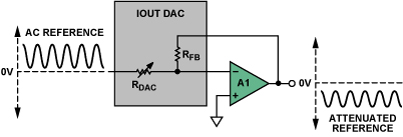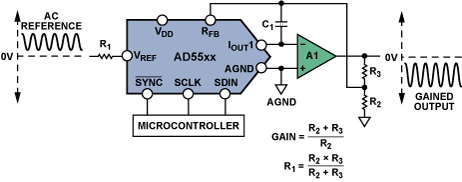# New High-Resolution Multiplying DACs Excel at Handling AC Signals

### Introduction

All digital-to-analog converters (DACs) provide an output proportional to the product of the digitally set gain and an applied reference voltage. A multiplying DAC differs from a fixed-reference DAC in that it can apply a high-resolution digitally set gain to a varying wideband analog signal. We discuss here resistance-ladder multiplying DACs and their inherent suitability for ac signal-processing applications.

### Basics

Since 1974, when Analog Devices introduced the world's first (10-bit) CMOS IC multiplying DAC, Analog Devices has been a leader in designing and producing multiplying DACs. Used with an amplifier having appropriate bandwidth, they employ a switched R-2R ladder and an on-chip feedback resistor to embody a simple method of adjusting the gain of an ac or varying dc reference input signal, using the DAC to replace the input and feedback resistors of a classic inverting op-amp stage (Figure 1). The digitally adjusted resistive ladder, with the on-chip feedback resistor, provides a gain (D/2n) proportional to the digital input, as if RDAC were a variable input resistor.Figure 1. Inverting gain configuration.

A burgeoning market has seen several generations of multiplying DACs, with increased resolution, accuracy, and speed; various digital storage functions; serial-communication options; reduced size and cost; and additional DACs per chip. The latest generation of multiplying DACs offer ideal building blocks for controlling the gain of varying dc or fast ac voltage signals.

The resistance (R-2R) ladder, used in an op-amp feedback circuit, provides a digitally controlled current that is translated to an output voltage by RFB. The amplifier provides this output at low impedance. The reference input has a constant resistance to ground, equal to R. Figure 2 shows the principle. In Figure 2a, one-half of the source current, VREF/R, is steered by switch S1 to either IOUT1, connected to the amplifier's negative input (at virtual ground), or to ground (often called IOUT2). One-half the remaining current is steered similarly by switch S2 ... and so on. If the switches are activated by a digital word, D (S1 is the MSB), the sum of the currents at IOUT1, flowing through RFB (=R), is D × 2–n × VREF/R. Important advantages of this configuration include minimization of transients, because the switches are switching between ground and virtual ground, and that RFB is matched on-chip to the ladder resistance, with excellent tracking over temperature.Figure 2. a) R-2R ladder principle. b) multiplying DAC, VOUT = 0 to −VREF.

The range of values given by the digital word, D, depends on the device used. Here are the ranges of D (first quadrant) for some Analog Devices multiplying DACs in the AD545x/AD554x families:

 8-bit AD5450 0 to 255 10-bit AD5451 0 to 1,023 12-bit AD5452 0 to 4,095 14-bit AD5453 0 to 16,383 16-bit AD554 3 0 to 65,535

Increasing the Gain

For applications in which the output voltage must be greater than VIN, gain can be added by following the DAC stage with an additional external amplifier; or it can be achieved in a single stage, by simply attenuating the feedback voltage, as shown in Figure 3. The approximation shown is valid for R2||R3<<RFB. R2 and R3 should have similar temperature coefficients, but if R2||R3 is small compared to RFB, they need not match the temperature coefficient of the DAC.Figure 3. Increasing the gain of a multiplying DAC.

### Positive Output

To generate a positive voltage output, an external inverting op amp circuit can be used to provide an additional inversion of either the input or the output. Because some multiplying DACs include uncommitted matched resistors (with tracking temperature coefficients), a positive output can be obtained simply by connecting an additional op amp (A2 in Figure 4)—which could be the companion op amp within a dual device.

If a differential output is required, two extra op amps are needed. Complete details can be found in Circuits from the Lab® CN-0143, www.analog.com/CN-0143.Figure 4. Multiplying DAC, VOUT = 0 to VREF. The AD5415, AD5405, AD5546/AD5556, AD5547/AD5557 include uncommitted resistors like those shown here.Figure 5. Single-ended to differential.

### Stability Issues

An important component shown in Figure 2 and Figure 3 is the compensation capacitor (C1). The output capacitance of the ladder, plus the amplifier's input capacitance and any strays, introduces a pole into the open-loop response—which can cause ringing or instability when the loop is closed. To compensate for this, an external feedback capacitor, C1, is usually connected in parallel with the internal RFB of the DAC. If the value of C1 is too small, it can produce overshoot or ringing at the output, while too large a value can unduly reduce the system bandwidth. Since the internal output capacitance of the DAC varies with code, it is difficult to fix a precise value for C1. The value is best approximated according to the equation:

where GBW is the small signal unity-gain bandwidth product of the op amp and CO is the output capacitance of the DAC.

### Key M-DAC Specifications for Signal Conditioning

Multiplying Bandwidth: The reference-input frequency at which the gain is –3 dB. For a given device, it is a function of amplitude and the choice of compensation capacitance. Figure 6 shows multiplying bandwidth plots for the AD5544, AD5554, or AD545x current-output DACs, which can multiply signals up to 12 MHz. The 350-MHz bandwidth of the accompanying low-power AD8038 op amp ensures that the op amp introduces insignificant dynamic errors on this scale.Figure 6. Multiplying bandwidth.

Analog Total Harmonic Distortion (THD):A mathematical representation of the harmonic content in the multiplied waveform signal. It is approximated by the log ratio of the rms sum of the first four harmonics (V2, V3, V4, and V5) of the DAC output to the fundamental value, V1, shown in Figure 7, and given by the equation:Figure 7. Harmonic distortion components.

Multiplying Feedthrough Error:The error due to capacitive feedthrough from the reference input to the DAC output, when the digital input to the DAC is all 0s. Ideally with each bit that is dropped, the gain is reduced by 6 dB, all the way down to the least significant bit, DB0 (Figure 8). However, for the lower bits the capacitive feedthrough affects the gain at higher frequencies. This can be seen by the flat lines tailing upwards for the lower bits. For example, at DB2 for a 14-bit DAC, the ideal gain should be –72 dB at all frequencies, but because of feedthrough the actual gain is –66 dB at 1 MHz.Figure 8. Multiplying feedthrough error.

### Choosing the Correct Op Amp

Multiplying-DAC circuit performance is strongly dependent on the ability of the selected op amp to maintain the voltage null at the ladder output and perform the current-to-voltage conversion. For best dc accuracy, it is important to select an operational amplifier with low offset voltage and bias current so as to keep errors commensurate with the DAC's resolution. Detailed op amp specifications are included in device data sheets.

For applications where the reference input is a relatively high speed signal, a wide-bandwidth, high-slew-rate op amp is required to avoid degrading the signal. The gain-bandwidth of an op-amp circuit is limited by the impedance level of the feedback network and the gain configuration. To determine what GBW is required, a useful guideline is to select an op amp with a –3-dB bandwidth that is 10 times the frequency of the reference signal.

The slew-rate specification of the op amp must be considered in order to limit distortion of large high-frequency signals. For the AD54xx and AD55xx families, an op amp with a slew rate of 100 V/µs is generally sufficient.

Table 1 provides a selection of operational amplifiers that are useful for multiplying applications

 Part Number Supply Voltage (V) BW (–3-dB) (MHz) Slew Rate (V/µs) VOS (Max) (µV) IB (Max) (nA) Package(s) AD8065 5 to 24 145 180 1500 0.006 SOIC-8, SOT-23-5 AD8066 5 to 24 145 180 1500 0.006 SOIC-8, MSOP-8 AD8021 5 to 24 490 120 1000 10,500 SOIC-8, MSOP-8 AD8038 3 to 12 350 425 3000 750 SOIC-8, SC70-5 ADA4899 5 to 12 600 310 35 100 LFCSP-8, SOIC-8 AD8057 3 to 12 325 1000 5000 500 SOT-23-5, SOIC-8 AD8058 3 to 12 325 850 5000 500 SOIC-8, MSOP-8 AD8061 2.7 to 8 320 650 6000 350 SOT-23-5, SOIC-8 AD8062 2.7 to 8 320 650 6000 350 SOIC-8, MSOP-8 AD9631 ±3 to ±6 320 1300 10,000 7000 SOIC-8, PDIP-8

### Finding the Right DAC

For a table of digital-to-analog converters, where M-DACs can be found, visit www.analog.com/en/products/digital-to-analog-converters.html.

### Conclusion

In the nearly 40 years of innovation since the introduction of the first CMOS M-DAC, a number of generations of devices have become available, with many new features, improved performance, and radical reductions in cost and size. Among the more recent improvements to our portfolio of high-resolution 14-bit/16-bit current-output AD55xx DACs are:

• Improved integral nonlinearity (INL), ±1 LSB
• Reduced analog THD and multiplying feedthrough—and wider multiplying bandwidths
• Reduced THD for digital signals; reduced midscale glitch and digital feedthrough for variable-reference (dc) applications.

### Further Reading

1. Kester, Walt, The Data Conversion Handbook (2005) Newnes (Elsevier).